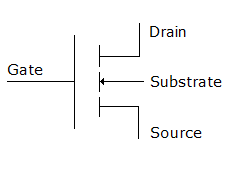# Electronics and Communication Engineering - Electronic Devices and Circuits

31.
When a ferromagnetic substance is magnetised, there are small changes in its dimensions. This phenomenon is called
hysteresis
magnetostriction
diamagnetism
bipolar relaxation
Explanation:
No answer description is available. Let's discuss.

32.
Gold is often diffused into silicon PN junction devices to
increase the recombination rate
reduce the recombination rate
make silicon a direct gap semiconductor
make silicon semimetal
Explanation:
No answer description is available. Let's discuss.

33.
In the figure shows the circuits symbol ofFET
PMOSFET
CMOSFET
NMOSFET
Explanation:
No answer description is available. Let's discuss.

34.

Assertion (A): FET is a unipolar device.

Reason (R): BJT is bipolar device.

Both A and R are true and R is correct explanation of A
Both A and R are true but R is not a correct explanation of A
A is true but R is false
A is false but R is true